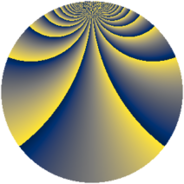# Properties

 Label 2001.2.bgLevel $2001$ Weight $2$ Character orbit 2001.bg Rep. character $\chi_{2001}(41,\cdot)$ Character field $\Q(\zeta_{44})$ Dimension $4720$ Sturm bound $480$

# Related objects

## Defining parameters

 Level: $$N$$ $$=$$ $$2001 = 3 \cdot 23 \cdot 29$$ Weight: $$k$$ $$=$$ $$2$$ Character orbit: $$[\chi]$$ $$=$$ 2001.bg (of order $$44$$ and degree $$20$$) Character conductor: $$\operatorname{cond}(\chi)$$ $$=$$ $$2001$$ Character field: $$\Q(\zeta_{44})$$ Sturm bound: $$480$$

## Dimensions

The following table gives the dimensions of various subspaces of $$M_{2}(2001, [\chi])$$.

Total New Old
Modular forms 4880 4880 0
Cusp forms 4720 4720 0
Eisenstein series 160 160 0

## Trace form

 $$4720q - 18q^{3} - 72q^{7} + O(q^{10})$$ $$4720q - 18q^{3} - 72q^{7} - 20q^{10} - 16q^{12} - 6q^{15} + 312q^{16} - 16q^{18} - 28q^{19} - 44q^{21} - 400q^{24} - 512q^{25} + 36q^{27} + 4q^{30} - 52q^{31} - 128q^{36} - 52q^{37} - 78q^{39} - 12q^{40} + 44q^{43} - 40q^{45} - 184q^{46} + 32q^{48} - 528q^{49} - 136q^{52} + 36q^{54} - 64q^{55} - 248q^{58} - 58q^{60} - 4q^{61} + 212q^{66} + 2q^{69} - 48q^{70} + 2q^{72} - 72q^{73} - 42q^{75} - 52q^{76} - 300q^{78} + 68q^{79} - 132q^{81} - 40q^{82} + 226q^{84} - 24q^{85} + 226q^{87} + 216q^{88} + 34q^{90} - 32q^{94} + 12q^{97} - 150q^{99} + O(q^{100})$$

## Decomposition of $$S_{2}^{\mathrm{new}}(2001, [\chi])$$ into newform subspaces

The newforms in this space have not yet been added to the LMFDB.